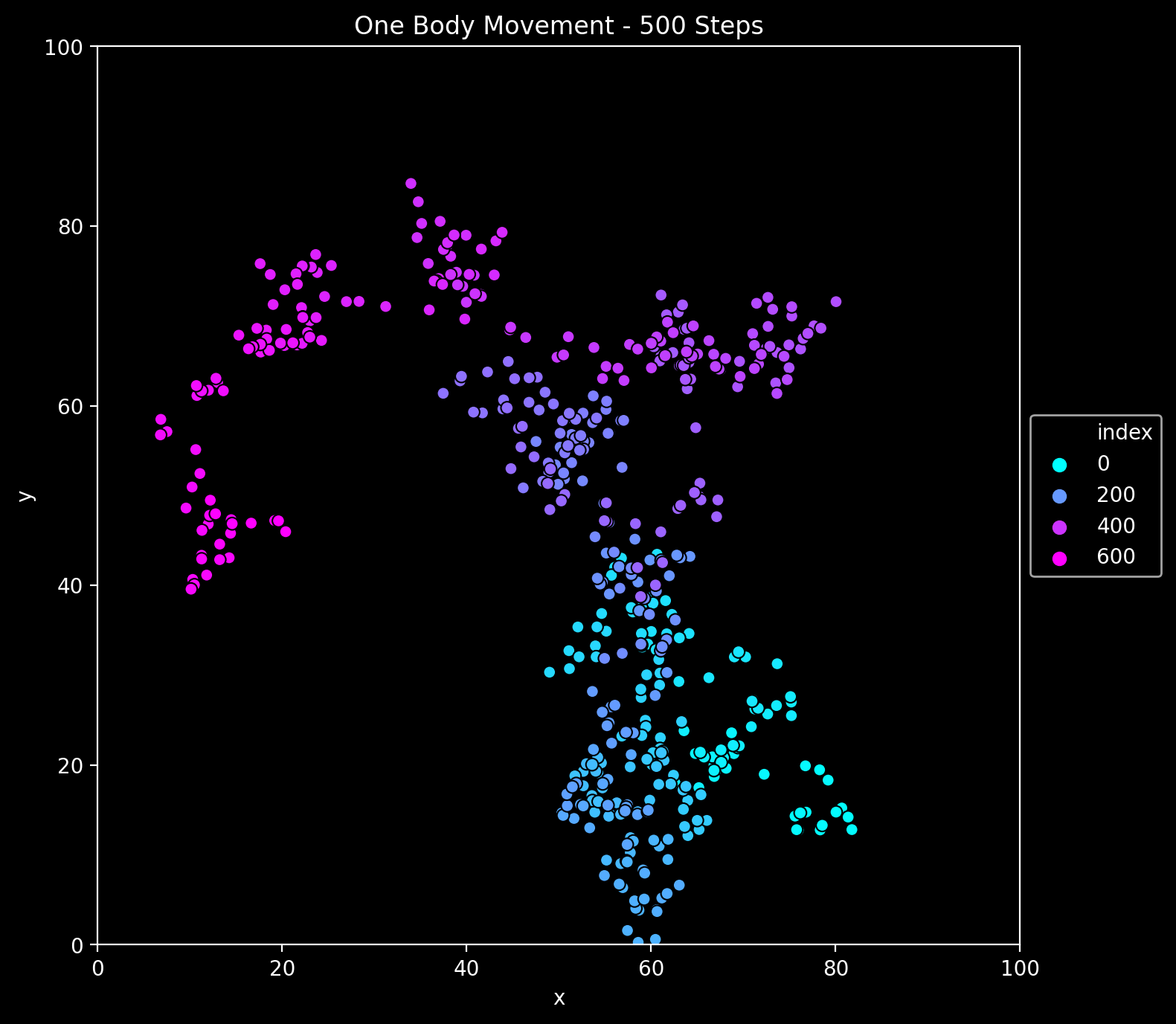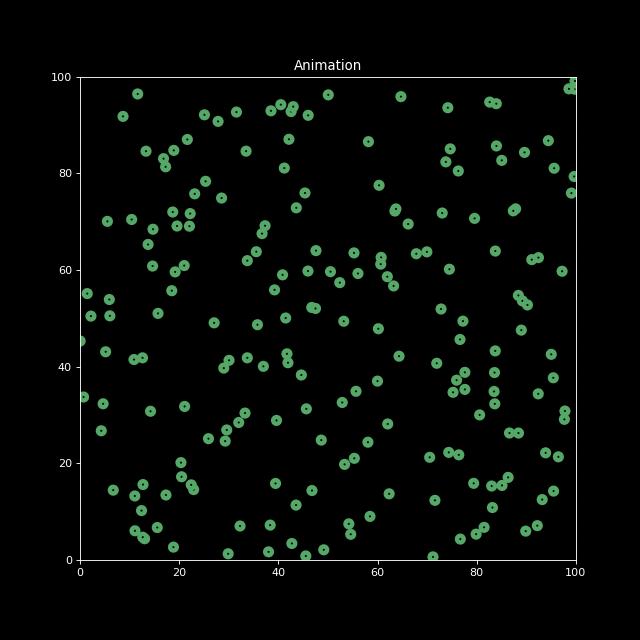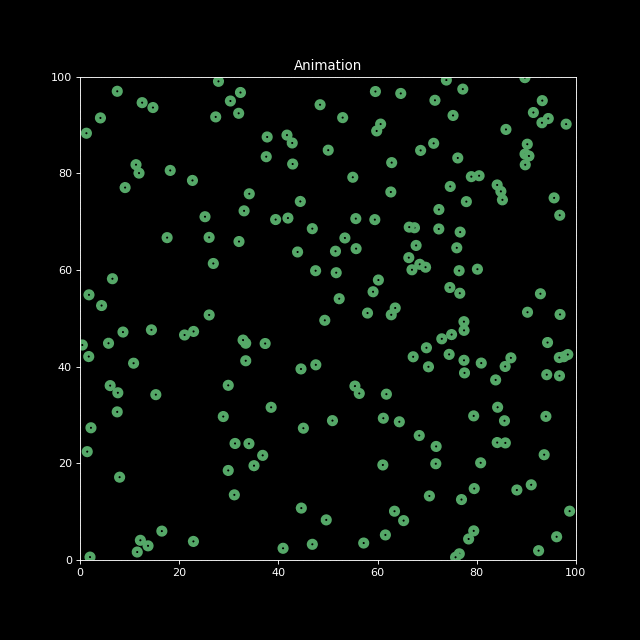This post is motivated by the tweet:

This was a perfect excuse to explore animations with Matplotlib.

Warning: This notebook does not aim to explain/predict anything related with covid19 (I leave that to the experts!). Please take this as a programming/data-viz exercise.

## Prepare Notebook

import numpy as np
import pandas as pd

import matplotlib.pyplot as plt
import seaborn as sns
plt.style.use(['dark_background'])
sns_c = sns.color_palette(palette='deep')
%matplotlib inline

from pandas.plotting import register_matplotlib_converters
register_matplotlib_converters()

from matplotlib.animation import FuncAnimation
from IPython.display import HTML

# Set global plot parameters.
plt.rcParams['figure.figsize'] = [8, 8]
plt.rcParams['figure.dpi'] = 80

## Model Definition

We define a class Body to represent each individual.

• Each body has a potion (x, y coordinates) inside a box of size $$L>0$$ (when it hits a boundary it rebounds).
• A body can move randomly (normal steps) within the box. We record each position after a movement.
• A body has a health status: sick or not sick.
• We assume that, if the body is sick, then it can infect other bodies with a probability (via Bernoulli distribution) $$\exp(-d/\tau)$$, where $$d$$ is the distance between two bodies and $$\tau$$ is a characteristic infection distance parameter.
• We record the health history on demand (not after every interaction).
L = 100

class Body():

def __init__(self, x0, y0, box_size=L, sigma=0.02, inf_dist=2):
self.box_size = box_size
self.x = x0
self.y = y0
self.sigma = sigma
self.loc_history = [(x0, y0)]
self.is_sick = False
self.health_history = [False]
self.inf_dist = inf_dist

self.loc_history.append((x, y))

self.health_history.append(x)

def get_infected(self):
self.is_sick = True

@staticmethod
def new_position(z, dz, box_size):
zhat = abs(z + dz)

if(zhat > box_size):
zhat = 2*box_size - zhat
return zhat

def move(self):
sigma = self.sigma * self.box_size
dx = np.random.normal(loc=0.0, scale=sigma)
dy = np.random.normal(loc=0.0, scale=sigma)

self.x = Body.new_position(z=self.x, dz=dx, box_size=self.box_size)
self.y = Body.new_position(z=self.y, dz=dy, box_size=self.box_size)

def distance(self, other):
return np.sqrt((self.x - other.x)**2 + (self.y - other.y)**2)

def interact(self, other):
dist = self.distance(other)
status = self.is_sick + other.is_sick

if ((status % 2) != 0):

if (self.is_sick):
prob = np.exp(-dist/self.inf_dist)
change_status = np.random.binomial(n=1, p=prob)
if (change_status):
other.get_infected()
else:
prob = np.exp(-dist/other.inf_dist)
change_status = np.random.binomial(n=1, p=prob)
if (change_status):
self.get_infected()

Let us see an example:

b1 = Body(0, 0)
b2 = Body(0.1, 0)
print('B1 is sick: ' + str(b1.is_sick))
print('B2 is sick: ' + str(b2.is_sick))
B1 is sick: False
B2 is sick: False
b2.get_infected()
b1.interact(b2)
print('B1 is sick: ' + str(b1.is_sick))
print('B2 is sick: ' + str(b2.is_sick))
B1 is sick: True
B2 is sick: True

Next let us define the Simulation class. This just makes a set of bodies interact in a box. The simulation starts wt just one sick body.

class Simulation():

def __init__(self, n_bodies, num, box_size=L, inf_dist=2):
self.box_size = box_size
self.n_bodies = n_bodies
self.num = num
self.bodies = [
Body(
x0=np.random.uniform(low=0.0, high=self.box_size),
y0=np.random.uniform(low=0.0, high=self.box_size),
box_size=self.box_size,
inf_dist=inf_dist
)
for i in range(0, self.n_bodies)
]
self.hist_len = 1

def run(self):
self.bodies.get_infected()
for i in range(self.num):
for j, b1 in enumerate(self.bodies):
for k, b2 in enumerate(self.bodies):
if (j < k):
b1.interact(b2)
[b.move() for b in self.bodies]
self.hist_len = self.hist_len + self.num

def animate(self):
fig, ax = plt.subplots()
ax.set(title='Animation', xlim=(0, self.box_size), ylim=(0, self.box_size))
scat = ax.scatter(x=[], y=[], c='black', linewidths=4)

def update(i):
x = [b.loc_history[i] for b in self.bodies]
y = [b.loc_history[i] for b in self.bodies]
x = np.array(x).reshape(-1, 1)
y = np.array(y).reshape(-1, 1)
scat.set_offsets(np.concatenate((x, y), axis=1))
scat.set_edgecolors(np.array([sns_c if b.health_history[i] else sns_c for b in self.bodies]))
return scat,

anim = FuncAnimation(fig, update, frames=self.hist_len, interval=200, blit=True)
return anim

## Results

First, let us plot a movement of one single body:

sim_1 = Simulation(n_bodies=1, num=500)
sim_1.run()

body_history_df = pd.DataFrame(sim_1.bodies.loc_history, columns=['x', 'y']).reset_index()

fig, ax = plt.subplots()
sns.scatterplot(x='x', y='y', hue='index', palette='cool', edgecolor='black', data=body_history_df, ax=ax)
ax.legend(loc='center left', bbox_to_anchor=(1, 0.5))
ax.set(title=f'One Body Movement - {sim_1.num} Steps', xlim=(0.0, L), ylim=(0.0, L))
plt.savefig('one_body.png', dpi=200, bbox_inches='tight');Next, let us generate an animation for n_bodies = 200 and num=100 (iterations).

sim = Simulation(n_bodies=200, num=100)
sim.run()

anim = sim.animate()
HTML(anim.to_jshtml())
anim.save('animation.gif', writer='imagemagick', fps=10)Finally, let us decrease the characteristic infection distance parameter to inf_dist=0.5. We indeed see the infection speed decreases.

sim2 = Simulation(n_bodies=200, num=100, inf_dist=0.5)
sim2.run()

anim2 = sim2.animate()
HTML(anim2.to_jshtml())
anim2.save('animation2.gif', writer='imagemagick', fps=10)## Remark: Animation with Matplotlib

This use case was a perfect setting to explore the animation module of Matplotlib.

• I believe it is very powerful, but non-trivial to start with. I found this stackoverflow thread very useful.

• I was not able to do a simple task: color the points based on the health status via scat.set_array(...). I had to color the edges of the points (if you manage to do it please let me know).

• It would be great to generate animations using seaborn.

• To save the animation as a gift you can run # !brew install imagemagick on a Jupyter cell.